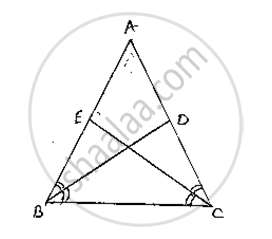Share
Notifications

View all notifications
Books Shortlist
Your shortlist is empty

# Bd and Ce Are Bisectors of ∠B and ∠C of an Isosceles δAbc with Ab = Ac. Prove that Bd = Ce. - CBSE Class 9 - Mathematics

Login
Create free account

Forgot password?
ConceptCongruence of Triangles

#### Question

BD and CE are bisectors of ∠B and ∠C of an isosceles ΔABC with AB = AC. Prove that BD = CE.

#### Solution

Given that ΔABC is isosceles with AB = AC and BD and CE are bisectors of ∠B and ∠C

We have to prove BD = C

Since AB = AC ⇒ ∠ABC = ∠ACB               …….(1)

[∵ Angles opposite to equal sides are equal]

Since BD and CE are bisectors of ∠B and ∠C

∠ABD = ∠DBC = ∠BCE = ECA =(∠B)/2=(∠C)/2`   …….(2)

Now,

Consider ΔEBC andΔDCB

∠EBC = ∠DCB       [∵ ∠B = ∠C ] from (1)

BC = BC                 [Common side]

∠BCE = ∠CBD        [ ∵ From (2)]

So, by ASA congruence criterion, we have  ΔEBC ≅ΔDCB
Now,

CE = BD         [∵  Corresponding parts of congruent triangles are equal]

or  BD = CE

∴Hence proved

Since AD || BC and transversal AB cuts at A and B respectively

∴∠DAO = ∠OBC   ……….(2) [alternate angle]

And similarly respectively AD || BC and transversal DC cuts at D ad C respectivaly

∴ ∠ADO = ∠OCB    ………(3) [alternate angle]

Since AB and CD intersect at O.

∴∠AOD = ∠BOC    [Vertically opposite angles]

Now consider ΔAOD and ΔBOD

∠DAO = ∠OBC     [∵ From (2)]

AD = BC               [ ∵ From (1)]

And ADO = OCB     [From (3)]

So, by ASA congruence criterion, we have

ΔAOD ≅ΔBO

Now,

AO = OB and DO = OC   [Corresponding parts of congruent triangles are equal]

⇒Lines AB and CD bisect at O.

∴Hence provedIs there an error in this question or solution?

#### APPEARS IN

Solution Bd and Ce Are Bisectors of ∠B and ∠C of an Isosceles δAbc with Ab = Ac. Prove that Bd = Ce. Concept: Congruence of Triangles.
S